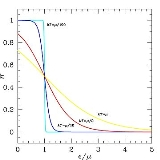xSemiconductorOverview

A semiconductor is a material with electrical conductivity due to electron flow (as opposed to ionic conductivity
Ionic conductivity
Ionic conduction is the movement of an ion from one site to another through defects in the crystal lattice of a solid. Ionic conduction is one aspect of current....

) intermediate in magnitude between that of a conductor
Electrical conductor
In physics and electrical engineering, a conductor is a material which contains movable electric charges. In metallic conductors such as copper or aluminum, the movable charged particles are electrons...

and an insulator. This means a conductivity roughly in the range of 103 to 10−8 siemens
Siemens (unit)
The siemens is the SI derived unit of electric conductance and electric admittance. Conductance and admittance are the reciprocals of resistance and impedance respectively, hence one siemens is equal to the reciprocal of one ohm, and is sometimes referred to as the mho. In English, the term...

per centimeter. Semiconductor materials are the foundation of modern electronics, including radio, computers, telephones, and many other devices.DiscussionsSemiconductor problem (we need soluton)